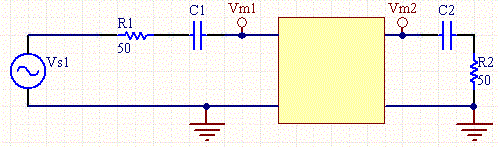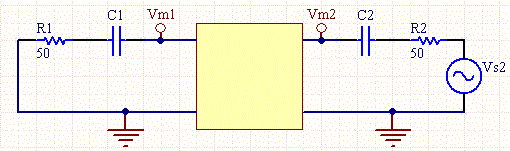# How to Measure S-Parameters

By Tim Daycounter

This tutorial explains how to measure S-Parameters without a fancy vector network analyzer (VNA). All you need are a scope and function generator capable of measuring and producing frequencies in the desired range.

When measuring S-Parameters on an active circuit you must include all of the biasing components as part of the two port circuit. Input frequencies need to be in the linear region of the circuit. Two 50 ohm resistors will be needed to act as terminators. For active circuits large DC blocking resistors will need to be used to couple the terminator resistors.

Figures 1 and 2 show the termination resistors, and the DC blocking caps if they are needed. If the circuit is symmetrical, then S11=S22 and S12=S21, so only one measurement need be done.Figure 1: Set up for measuring S-Parameter S11 and S12.Figure 2: Set up for measuring S-Parameter S22 and S21.

To make the measurement set the frequency of the function generator to the desired frequency, and measure the source voltage, and Vm1 and Vm2 for both configurations. The phase measurement is the hardest one to make, since it's hard to view small differences in phase on a scope. Then make the following calculations:

S11= 2*Vm1/Vs1-1

S12= 2Vm2/Vs1

S21= 2Vm1/Vs2

S22= 2*Vm2/Vs2-1

The hardest part is remember the correct polarity convention for the phase. Assume that source voltages have zero phase. Then measure the phase relative to the measured signal. If the phase of the measured signal lags (is to the right) then the phase should be negative. The voltage division is simple because, since the phase of the divisor is zero, you just need to divide magnitudes. To subtract 1 you need to convert to rectangular coordinates. S11 and S22 should always be less than 1 in magnitude, and for active circuits S12 and S21 can be greater than 1.

A good way to test out your S-Parameter measuring skills is to create a simple T circuit, where the Z parameters are easy to pick off. Then do the measurements and convert the S parameters to Z parameters using our S to Z parameter converter. The parameters should be reasonably close.